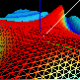# How to add 2 Mat objects of different type?Hi all, When I run the following code:

const Mat A( 10, 20, CV_32FC1, Scalar::all(CV_PI) );
const Mat B( A.size(), CV_8UC1, Scalar::all(10) );
Mat C( A.size(), A.type() );
C = A + B;


I get the run-time error:

    OpenCV Error: Bad argument (When the input arrays in add/subtract/multiply/divide
functions have different types, the output array type must be explicitly specified) in
arithm_op, file /usr/local/src/OpenCV/modules/core/src/arithm.cpp, line 1269


So, is there a way to explicitly specify the output array type of A+B as float? Thanks

edit retag close merge delete

Sort by » oldest newest most votedI think it impossible using + operator. You can:

• Use convert to float

const Mat A( 10, 20, CV_32FC1, Scalar::all(CV_PI) );
const Mat B( A.size(), CV_8UC1, Scalar::all(10) );
Mat E(A.size(), A.type());
B.convertTo(E, CV_32FC1);
Mat C( A.size(), A.type() );
C = E+A;

• Per-element sum

const Mat A( 10, 20, CV_32FC1, Scalar::all(CV_PI) );
const Mat B( A.size(), CV_8UC1, Scalar::all(10) );
Mat C(A.size(), A.type());
for (int i = 0; i < A.rows; i++)
{
for (int j = 0; j < A.cols; j++)
{
C.at<float>(i,j) = A.at<float>(i,j)+B.at<uchar>(i,j);
}
}


or


const Mat A( 10, 20, CV_32FC1, Scalar::all(CV_PI) );
const Mat B( A.size(), CV_8UC1, Scalar::all(10) );
Mat C(A.size(), A.type());
for (int i = 0; i < A.rows; i++)
{
const float* Aptr = reinterpret_cast< const float*>(A.ptr(i));
const uchar* Bptr = reinterpret_cast< const uchar*>(B.ptr(i));
float* Cptr = reinterpret_cast< float*>(C.ptr(i));
for (int j = 0; j < A.cols; j++)
{
Cptr[j] = Aptr[j]+Bptr[j];
}
}

more

Thank Alex Shishkov! Very excellent answer!

I have one comment only. For the first method of conversion, matrices C and E do not need to be initialized explicitly.So it is also OK to be like this:

const Mat A( 10, 20, CV_32FC1, Scalar::all(CV_PI) );
const Mat B( A.size(), CV_8UC1, Scalar::all(10) );
Mat C,E;
B.convertTo(E, CV_32FC1);
C = E+A;

more

2

My 2 cents to the 3d version Alex suggested:

const float* Aptr = A.ptr<float>(i);

is better than

const float* Aptr = reinterpret_cast< const float*>(A.ptr(i));

Official site

GitHub

Wiki

Documentation